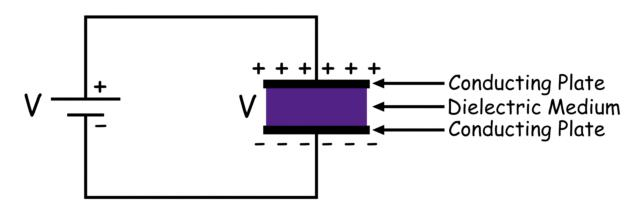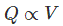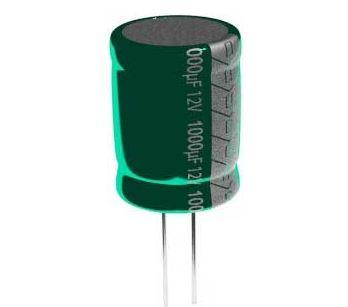# What is a Capacitor, And What is Capacitance?

Contents## What is a Capacitor?

Capacitors are one of three fundamental electronic components that form the foundation of a circuit – along with resistors and inductors. A capacitor in an electrical circuit behaves as a charge storage device. It holds the electric charge when we apply a voltage across it, and it gives up the stored charge to the circuit as when required.

The most basic construction of a capacitor consists of two parallel conductors (usually metallic plates) separated by a dielectric material.

When we connect a voltage source across the capacitor, the conductor (capacitor plate) attached to the positive terminal of the source becomes positively charged, and the conductor (capacitor plate) connected to the negative terminal of the source becomes negatively charged.

Because of the presence of dielectric in between the conductors, ideally, no charge can migrate from one plate to other.So, there will be a difference in charging level between these two conductors (plates). Therefore an electric potential difference appears across the plates.

The charge accumulation in the capacitor plates is not instantaneous rather it is gradually changing.

The voltage appears across the capacitor exponentially rises untill it becomes equal to that of the connected voltage source.

## What is Capacitance?

Now we understand that the charge accumulation in the conductors (plates) causes the voltage or potential difference across the capacitor. The quantity of charge accumulated in the capacitor for developing a particular voltage across the capacitor is referred to as the charge holding capacity of the capacitor.

We measure this charge accumulation capability of a capacitor in a unit called capacitance. The capacitance is the charge gets stored in a capacitor for developing 1 volt potential difference across it.

Hence, there is a direct relationship between the charge and voltage of a capacitor. The charge accumulated in the capacitor is directly proportional to the voltage developed across the capacitor.Where Q is the charge and V is the voltage.Here C is the constant of proportionality, and this is capacitance,

The capacitance depends upon three physical factors, and these are the active area of the capacitor conductor (plates), the distance between the conductors (plates) and permittivity of the dielectric medium.Here, ε is permittivity of the dielectric medium, A is the active area of the plate and d is the perpendicular distance between the plates.Want To Learn Faster? 🎓
Get electrical articles delivered to your inbox every week.
No credit card required—it’s 100% free.